# k-means clustering¶

k-means clustering partitions n observations into k clusters in which each observation belongs to the cluster with the nearest mean.

Here we're implementing a heuristic algorithm for k-means clustering.

We have N points that we want to partition into K clusters.

It works this way:

### Step 1: Initialize clusters¶

1. Pick K random points from the N points.
2. Assign each of the other N-K points to a cluster $C_i$ where "i" is the index of the closest point from the K points selected above.

### Step 2: Iterate on changing the clusters until convergence¶

Repeat until no changes:

1. For each cluster, compute its mean.
2. Iterate over all N points and assign each one to a cluster indexed by the closest mean.

This is displayed and inplemented below:

In :
import random
import numpy as np
import matplotlib.pyplot as plt

def plot_points(title, use_colors=True):
xs = [p for p in points]
ys = [p for p in points]
assert(len(xs) == N)
if use_colors:
colors = [(cc / float(K)) for cc in cluster]
plt.scatter(xs, ys, edgecolors='none', s=30, c=colors)
else:
plt.scatter(xs, ys, edgecolors='none', s=30)
plt.title(title)
plt.show()

# Number of points.
N = 720

# Number of clusters.
K = 6

points = []
for i in range(0, N):
x = random.randint(0, 100)
y = random.randint(0, 100)
points.append((x, y))

plot_points('Initial %d points which we\'ll split into %d clusters.' % (N, K), use_colors=False)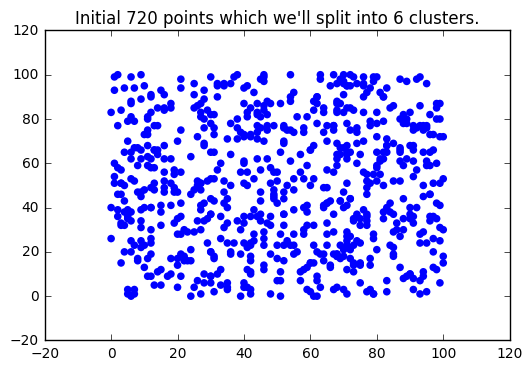In :
cluster = [-1] * N

indices = range(0, N)
random.shuffle(indices)

mean_point_idxs = indices[0: K]

for i in range(0, K):
cluster[ mean_point_idxs[i] ] = i

for idx in range(0, N):
if idx not in mean_point_idxs:
# Find closest.
ci = -1
mindistance = 1 << 30
for i in range(0, K):
cm = mean_point_idxs[i]
distance = (points[idx] - points[cm]) ** 2 + (points[idx] - points[cm]) ** 2
if distance < mindistance:
mindistance = distance
ci = i

cluster[idx] = ci

plot_points('Initial cluster assignment.')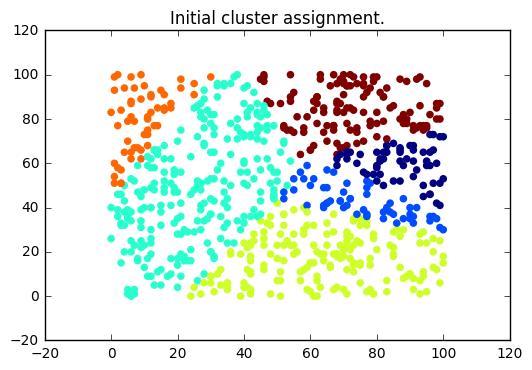In :
for iter in range(0, 50):
# Compute mean for every cluster.
sumx = [0.0] * K
sumy = [0.0] * K
totals =  * K
for i in range(0, N):
sumx[ cluster[i] ] += points[i]
sumy[ cluster[i] ] += points[i]
totals[ cluster[i] ] += 1

# Compute new means.
meanx =  * K
meany =  * K
for i in range(0, K):
meanx[i] = sumx[i] / totals[i]
meany[i] = sumy[i] / totals[i]

# Create new clusters.
new_cluster =  * N
for i in range(0, N):
mindist = 1 << 30
for j in range(0, K):
dist = (points[i] - meanx[j]) ** 2 + (points[i] - meany[j]) ** 2
if dist < mindist:
mindist = dist
cluster_idx = j

new_cluster[i] = cluster_idx

if cluster == new_cluster:
print 'DONE'
break
else:
moved = 0
for i in range(0, N):
if cluster[i] != new_cluster[i]: moved += 1
print 'MOVED =', moved

cluster = new_cluster

plot_points('Clusters after iteration %d' % (iter+1))

MOVED = 111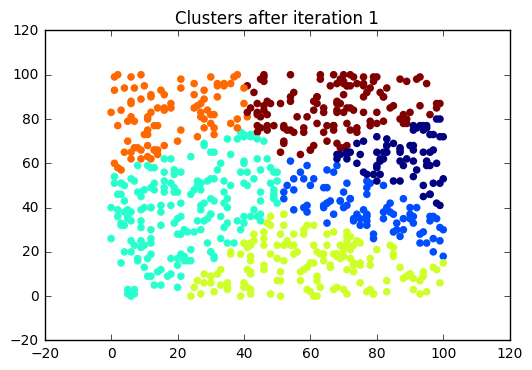MOVED = 66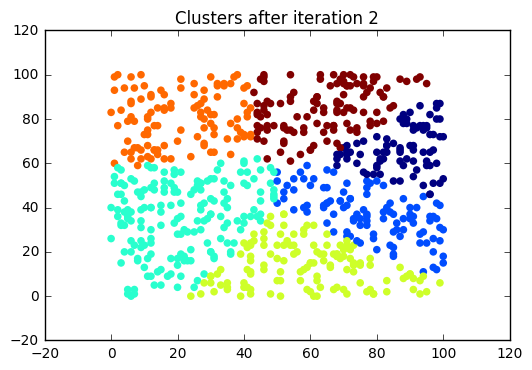MOVED = 36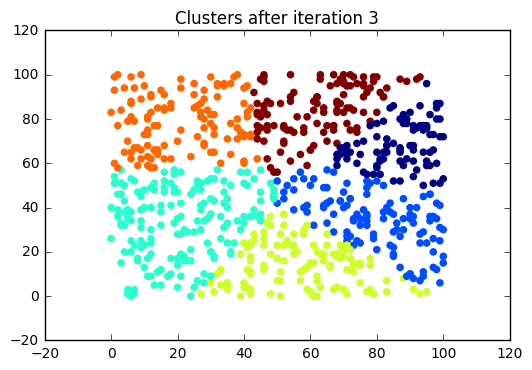MOVED = 28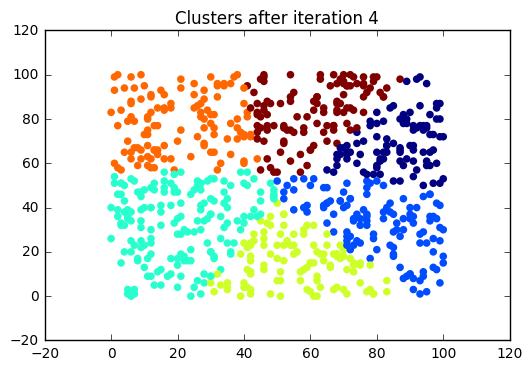MOVED = 29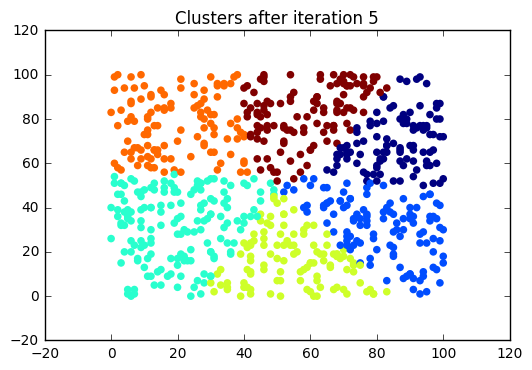MOVED = 25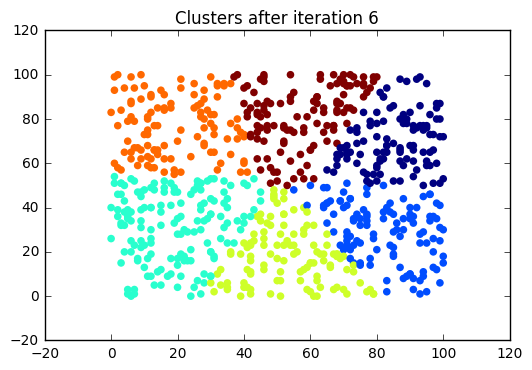MOVED = 21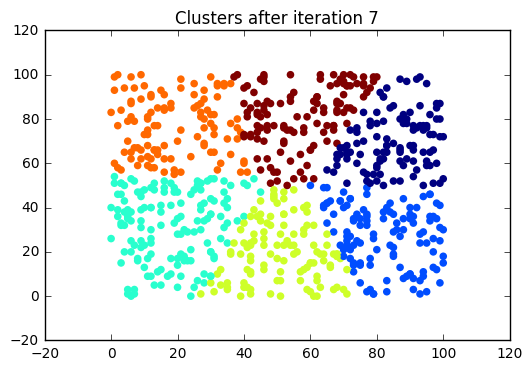MOVED = 21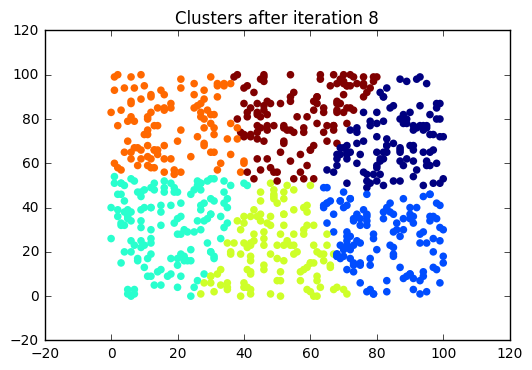MOVED = 11